Chapter 3 Class 10 Pair of Linear Equations in Two Variables

Class 10
Important Questions for Exam - Class 10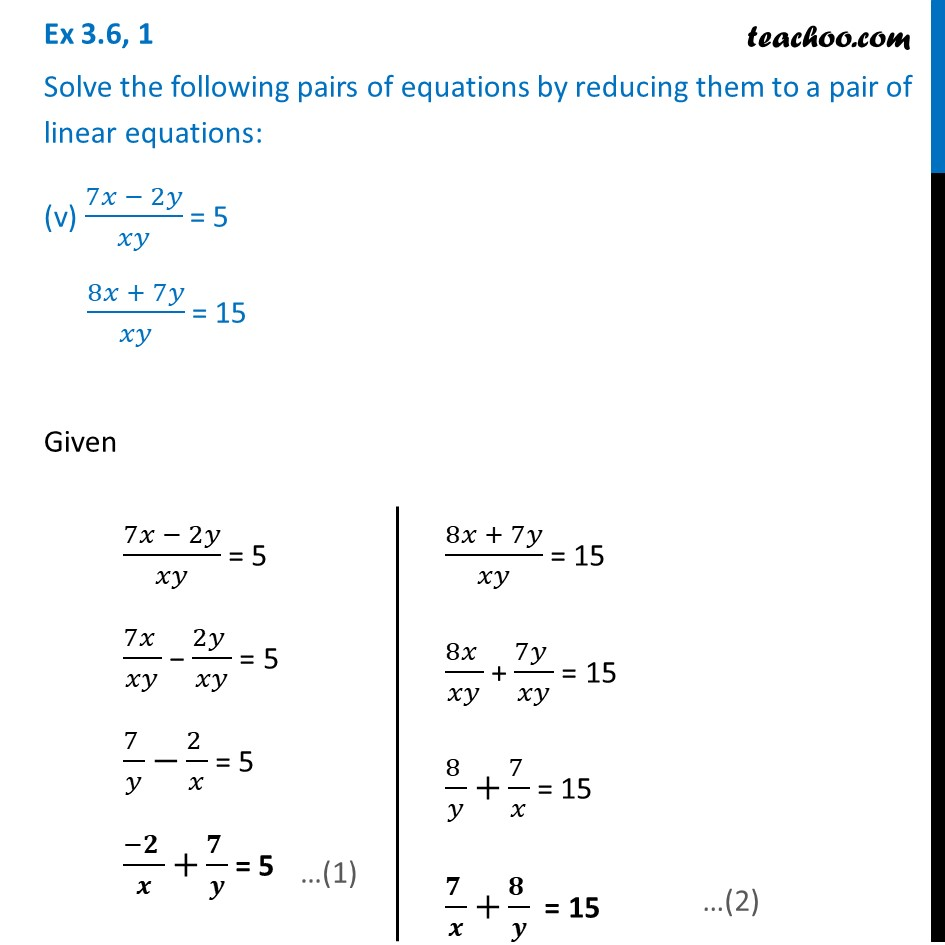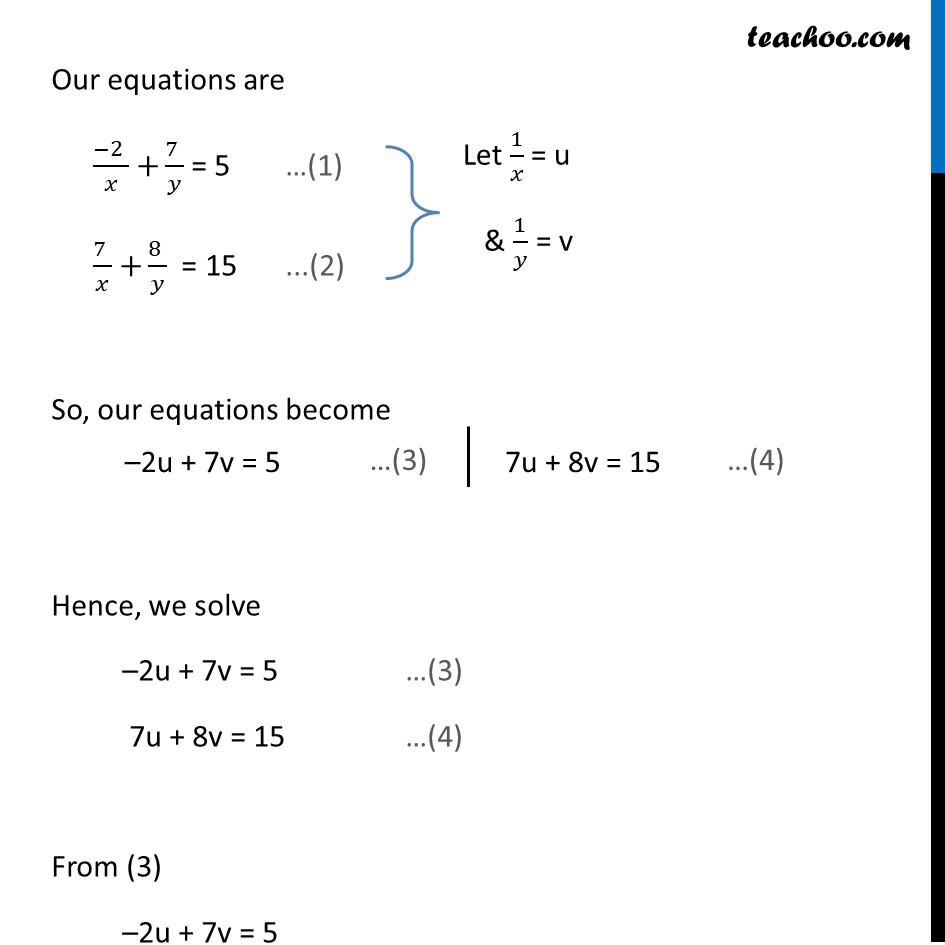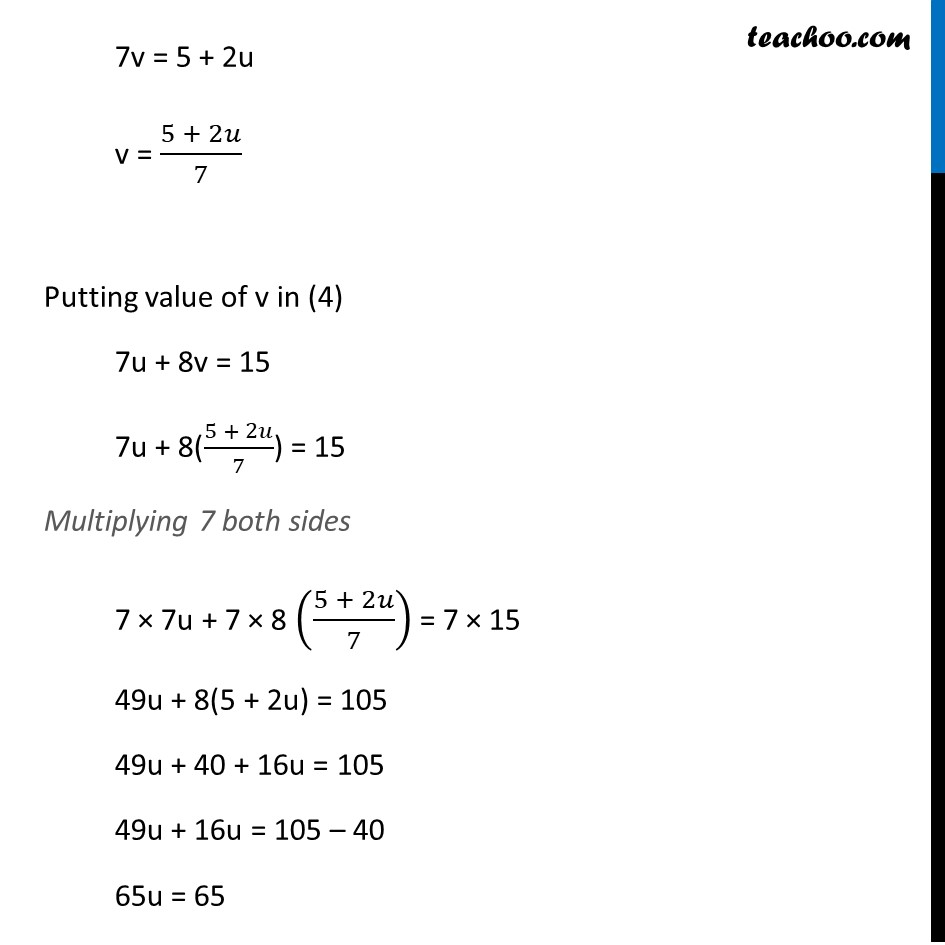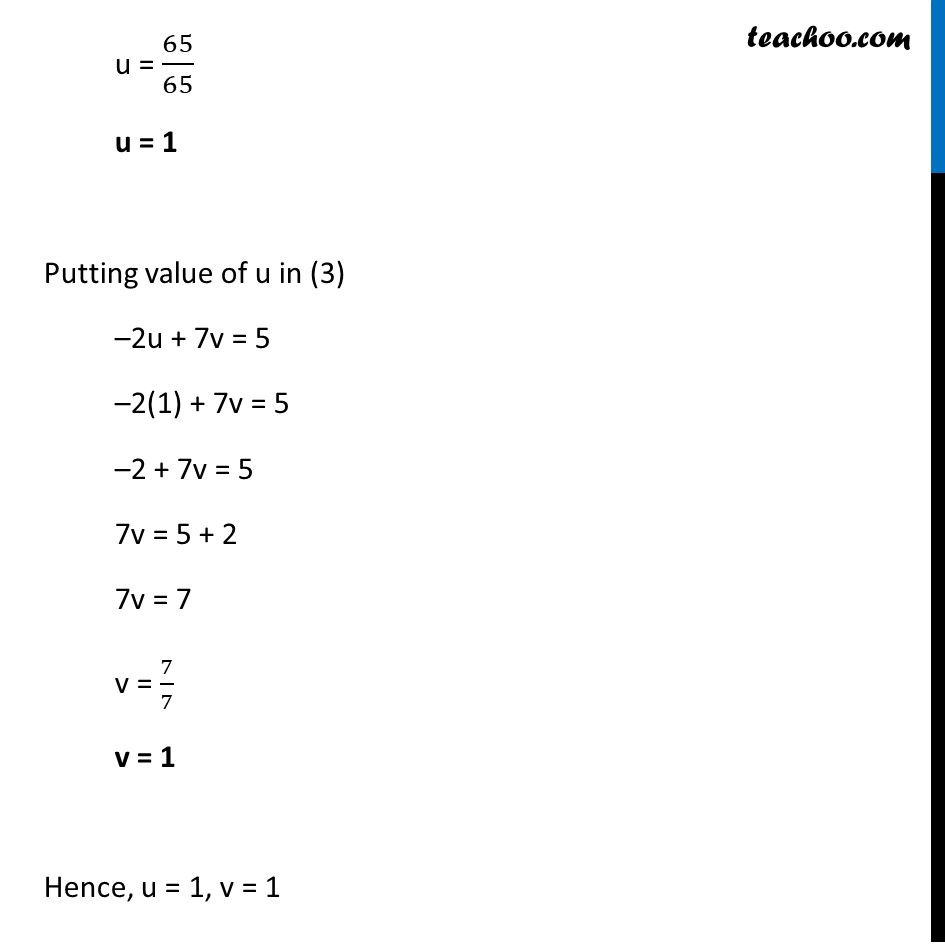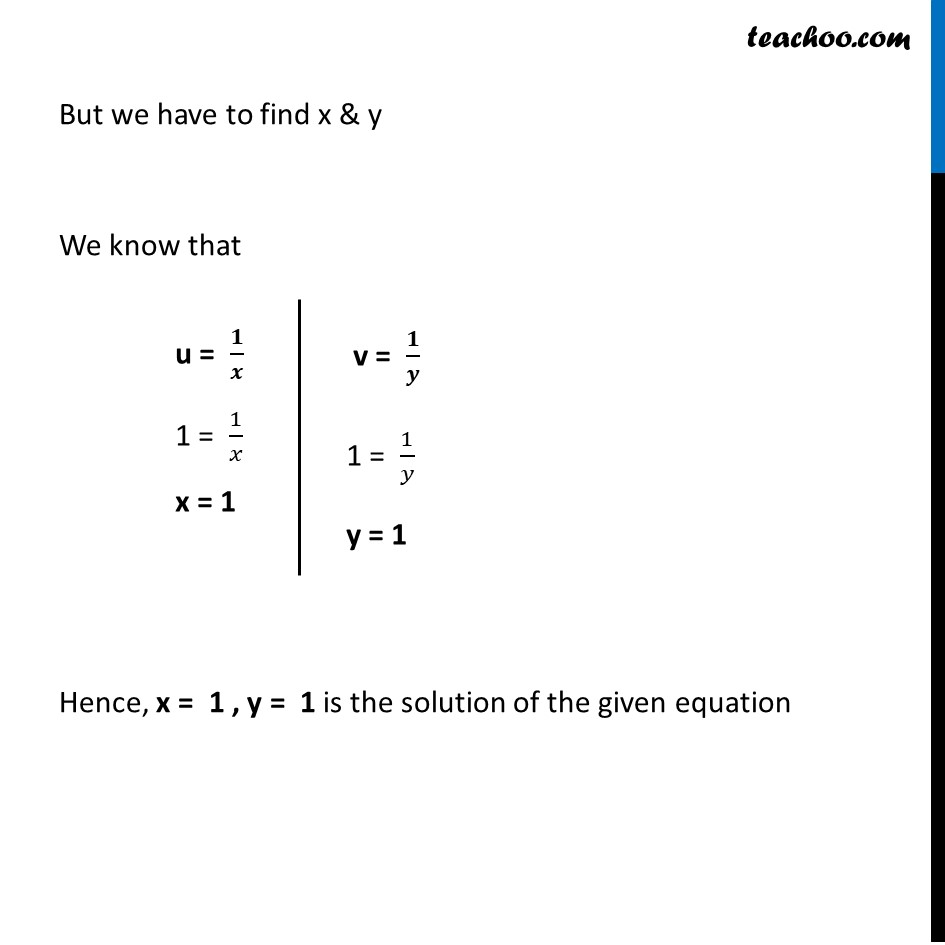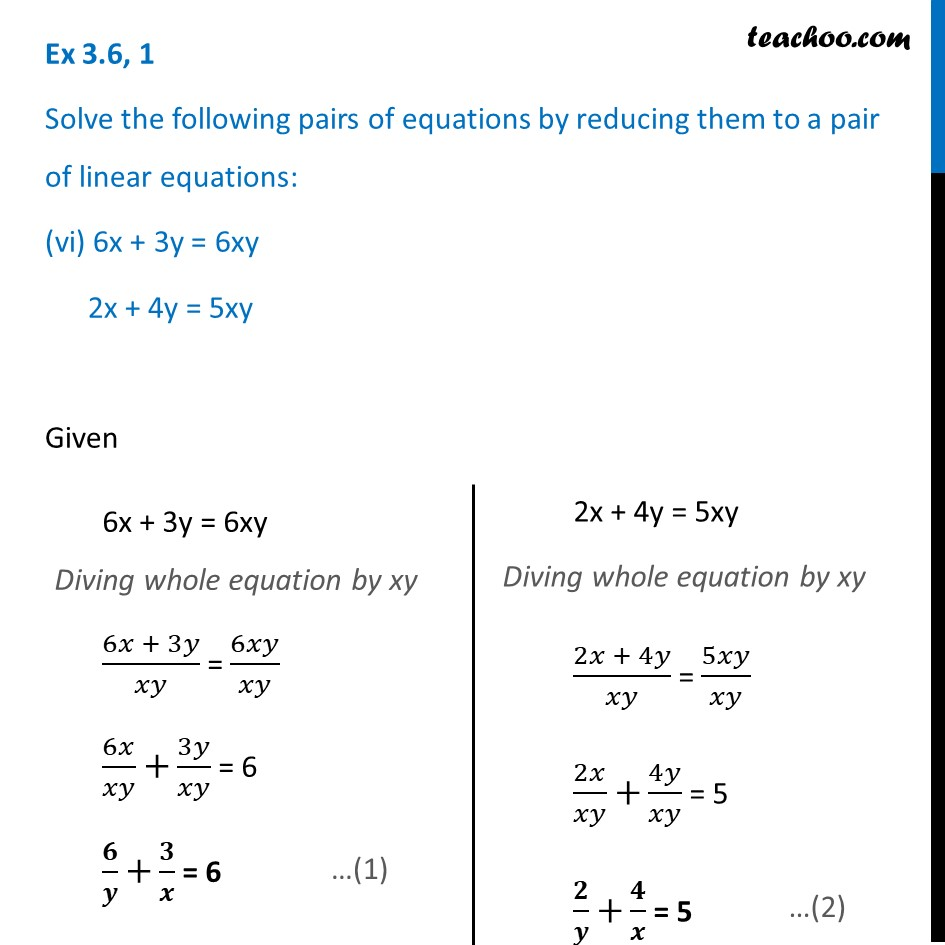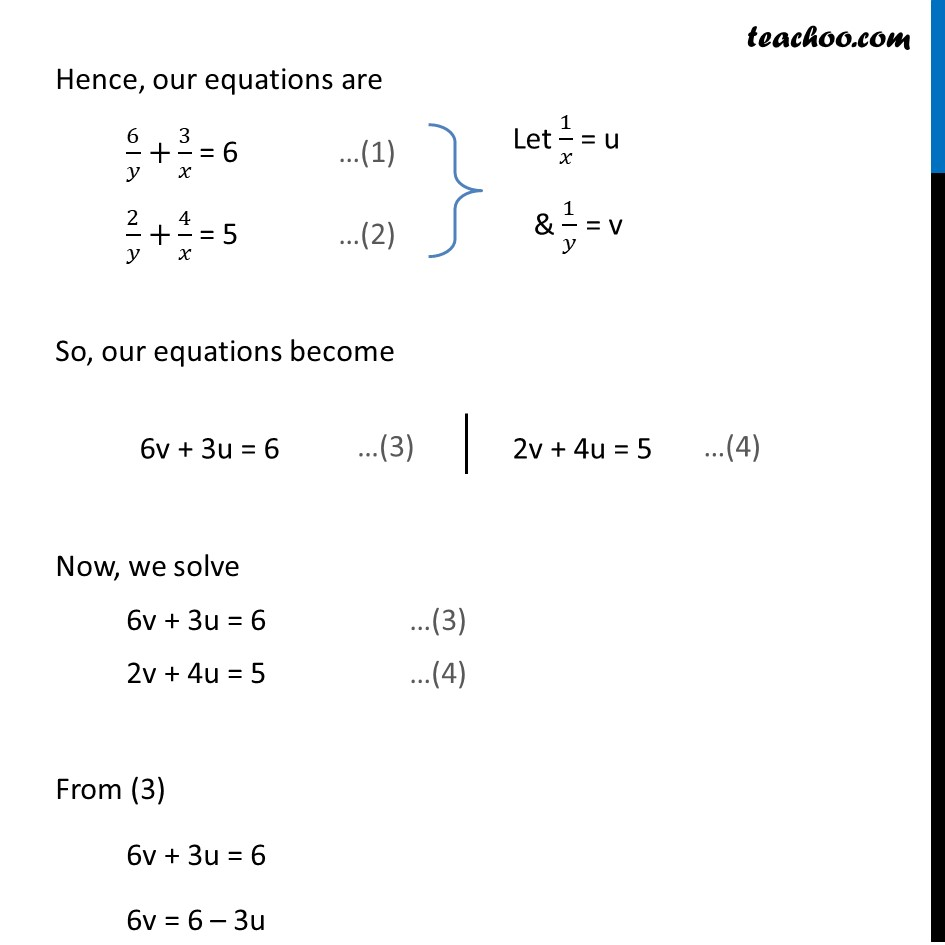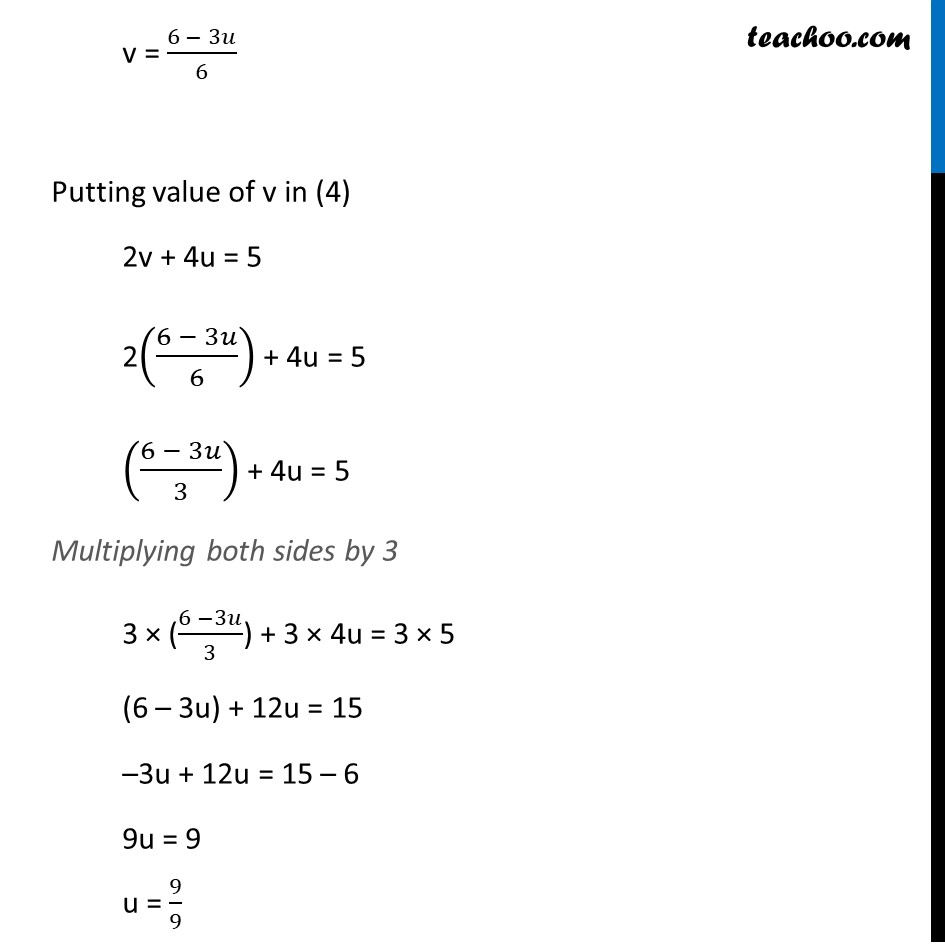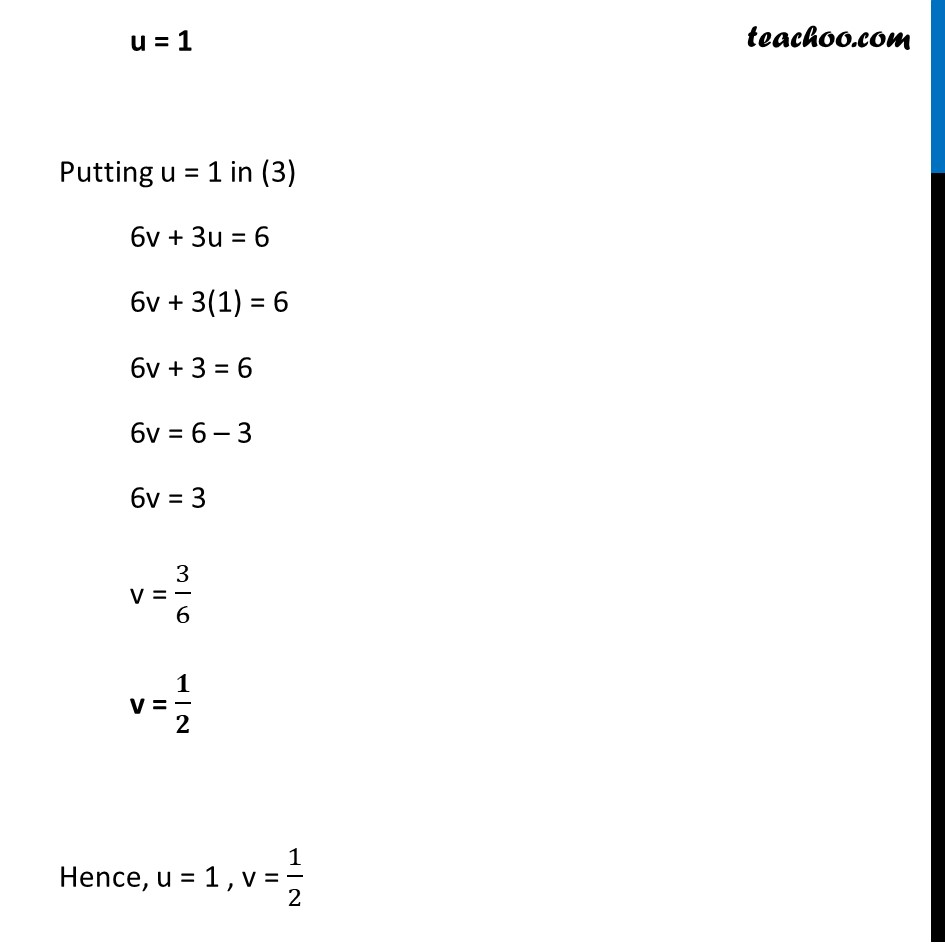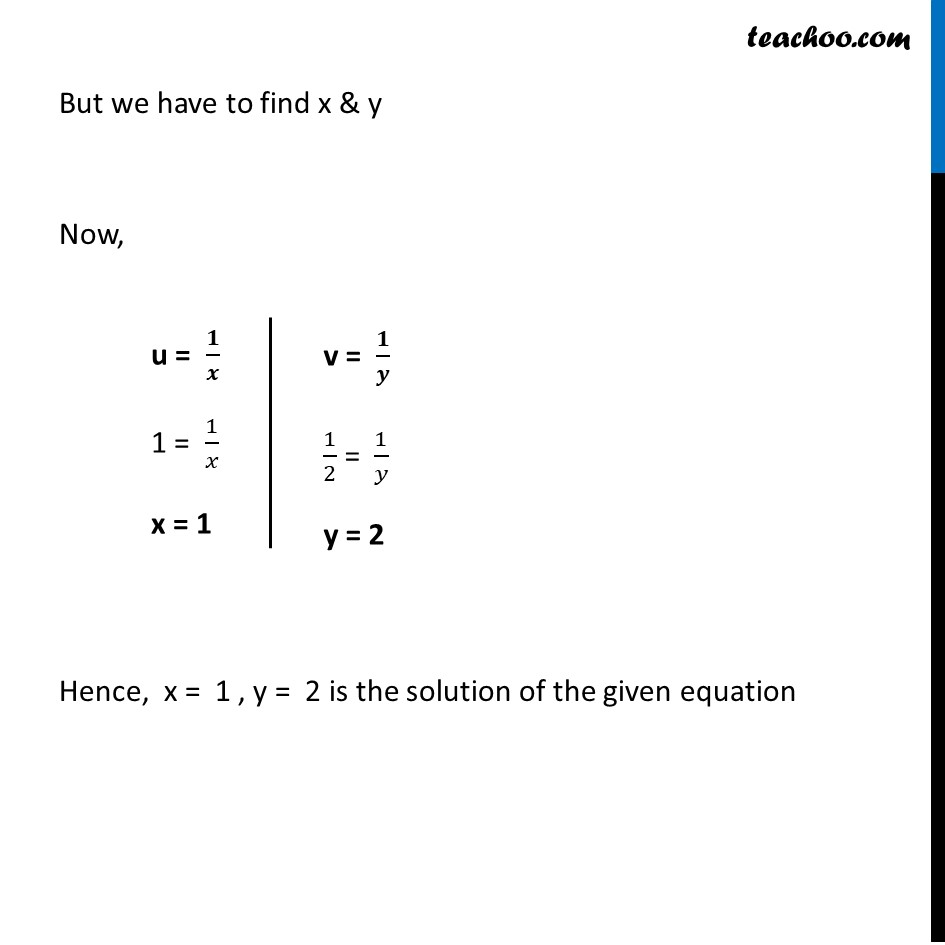Learn in your speed, with individual attention - Teachoo Maths 1-on-1 Class

### Transcript

Question 1 Solve the following pairs of equations by reducing them to a pair of linear equations: (v) (7𝑥 − 2𝑦)/𝑥𝑦 = 5 (8𝑥 + 7𝑦)/𝑥𝑦 = 15 Given (7𝑥 − 2𝑦)/𝑥𝑦 = 5 (7𝑥 )/𝑥𝑦 − (2𝑦 )/𝑥𝑦 = 5 (7 )/𝑦 −(2 )/𝑥 = 5 (−𝟐 )/𝒙 +(𝟕 )/𝒚 = 5 (8𝑥 + 7𝑦)/𝑥𝑦 = 15 (8𝑥 )/𝑥𝑦 + (7𝑦 )/𝑥𝑦 = 15 (8 )/𝑦 +(7 )/𝑥 = 15 (𝟕 )/𝒙 +(𝟖 )/𝒚 = 15 Our equations are (−2 )/𝑥 +(7 )/𝑦 = 5 …(1) (7 )/𝑥 +(8 )/𝑦 = 15 ...(2) So, our equations become –2u + 7v = 5 7u + 8v = 15 Hence, we solve –2u + 7v = 5 …(3) 7u + 8v = 15 …(4) From (3) –2u + 7v = 5 7v = 5 + 2u v = (5 + 2𝑢)/7 Putting value of v in (4) 7u + 8v = 15 7u + 8((5 + 2𝑢)/7) = 15 Multiplying 7 both sides 7 × 7u + 7 × 8 ((5 + 2𝑢)/7) = 7 × 15 49u + 8(5 + 2u) = 105 49u + 40 + 16u = 105 49u + 16u = 105 – 40 65u = 65 u = 65/65 u = 1 Putting value of u in (3) –2u + 7v = 5 –2(1) + 7v = 5 –2 + 7v = 5 7v = 5 + 2 7v = 7 v = 7/7 v = 1 Hence, u = 1, v = 1 But we have to find x & y We know that u = 𝟏/𝒙 1 = 1/𝑥 x = 1 v = 𝟏/𝒚 1 = 1/𝑦 y = 1 Hence, x = 1 , y = 1 is the solution of the given equation Question 1 Solve the following pairs of equations by reducing them to a pair of linear equations: (vi) 6x + 3y = 6xy 2x + 4y = 5xy Given 6x + 3y = 6xy Diving whole equation by xy (6𝑥 + 3𝑦)/𝑥𝑦 = 6𝑥𝑦/𝑥𝑦 6𝑥/𝑥𝑦 +3𝑦/𝑥𝑦 = 6 𝟔/𝒚 +𝟑/𝒙 = 6 2x + 4y = 5xy Diving whole equation by xy (2𝑥 + 4𝑦)/𝑥𝑦 = 5𝑥𝑦/𝑥𝑦 2𝑥/𝑥𝑦 +4𝑦/𝑥𝑦 = 5 𝟐/𝒚 +𝟒/𝒙 = 5 Hence, our equations are 6/𝑦 +3/𝑥 = 6 …(1) 2/𝑦 +4/𝑥 = 5 …(2) So, our equations become 6v + 3u = 6 2v + 4u = 5 Now, we solve 6v + 3u = 6 …(3) 2v + 4u = 5 …(4) From (3) 6v + 3u = 6 6v = 6 – 3u v = (6 − 3𝑢)/6 Putting value of v in (4) 2v + 4u = 5 2((6 − 3𝑢)/6) + 4u = 5 ((6 − 3𝑢)/3) + 4u = 5 Multiplying both sides by 3 3 × ((6 −3𝑢)/3) + 3 × 4u = 3 × 5 (6 – 3u) + 12u = 15 –3u + 12u = 15 – 6 9u = 9 u = 9/9 u = 1 Putting u = 1 in (3) 6v + 3u = 6 6v + 3(1) = 6 6v + 3 = 6 6v = 6 – 3 6v = 3 v = 3/6 v = 𝟏/𝟐 Hence, u = 1 , v = 1/2 But we have to find x & y Now, u = 𝟏/𝒙 1 = 1/𝑥 x = 1 v = 𝟏/𝒚 1/2 = 1/𝑦 y = 2 Hence, x = 1 , y = 2 is the solution of the given equation